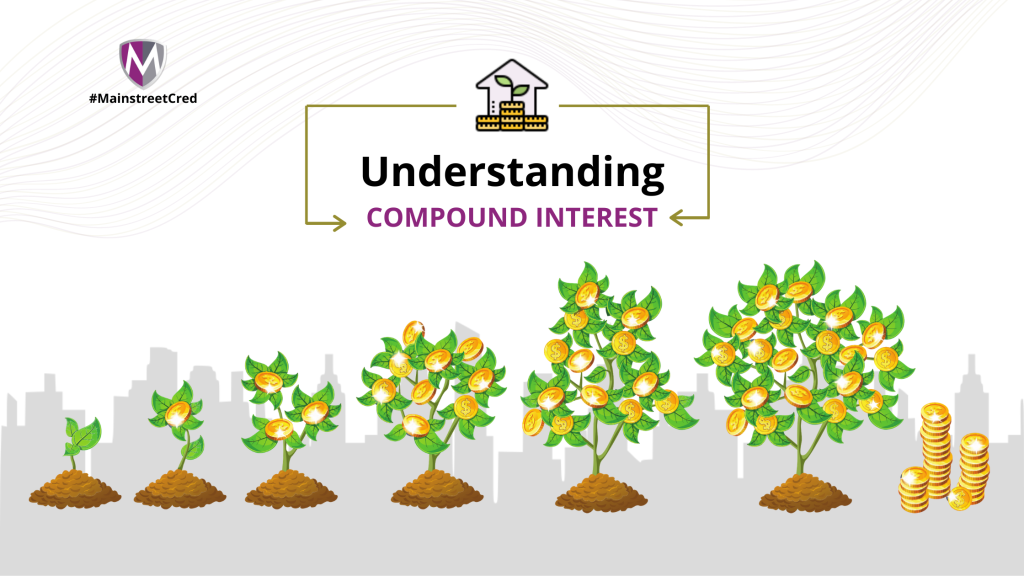# Compound Interest Investments

Compound interest (or compounding interest) is interest calculated on the initial principal (Initial Investment) plus interest accrued in previous period. To think of it simply, a compound interest is interest on interest. When an investment is made and it generates interest, even more interest is incurred on the generated interest.

However, banks work with Simple Interest. Simple interest is determined by multiplying the daily interest rate by the principal by the number of days the money is saved.

If a person saves ₦100,000 at an interest if 10%, the interest after 1 year will be 10% of 100,000 which is 10,000.

It is important to note that with Nigerian banks, interest only applies when a company doesn’t withdraw more than 3 times. By the 4th withdrawal, the interest is forfeited.

## Example of Compound Interest for a Salary Earner

Anita invests ₦100,000 in the Mainstreet Capital Flexi Savings Product monthly which for the period she invested, has a stable annual interest rate of 10% and a quarterly Compounding of investments.

The interest for the year on ₦100,000 is ₦10,000

When an interest rate is 10%, it means that everyday, the amount earned on that investment is 10% of that investment divided by 365 (The number of days in the year).

S0, ₦10,000/365 = ₦27.40/day.

After a month (30 days), Anita earns 27.397 * 30 = ₦821.92/month

### January

Initial Investment = ₦100,000 inflow

Principal = ₦100,000

Interest = ₦821.92

### February

₦100,000 inflow

New Principal = ₦100,000 + ₦100,000 = ₦200,000

Interest of 10% is 20,000

Daily Interest of 20,000/365 = ₦54.79

Monthly Interest = ₦54.79 * 30 = ₦1,650.59

### March

₦100,000 inflow

New Principal = ₦300,000

Monthly Interest on ₦300,000 = ₦2,465.75

### Quarterly Compounding

After the first quarter, the Interest (which will roll over) is ₦821.92 ­+ ₦1,650.59 + ₦2,465.75 = ₦4938.26

For the next month, ₦4938.26 will be added to the principal. This is what is meant by “Compounding”

### April

₦100,000 inflow

New Principal = ₦300,000 + ₦100,000 + ₦4938.26 = ₦404,938.26

Monthly Interest on ₦404,938.26 = ₦3,328.26

## The Potential of Compound Interest

As seen in the table above, it starts out seeming irrelevant in the first year and ends with a difference of ₦1475.76. The beauty of compound interest begins to manifest after the second year of investing. An unbelievable ratio emerges, growing exponentially with each passing year.

By the 3rd year, the Compound Interest Investor would be earning almost twice what the simple interest investor would.

By the 4th year, the Compound Interest Investor would be earning 2.61 times what the simple interest investor would.

By the 6th year, the Compound Interest Investor would be earning 4 times what the simple interest investor would.

By the 10th year, the Compound Interest Investor would be earning almost 7 times what the simple interest investor would.

### WORK YOUR WAY INTO FINANCIAL FREEDOM BY TAKING THIS FIRST STEP

Open an account here

or

Talk to our Asset Management Advisors here

## Compound Interest and Time

Another example- Let’s take 2 women for example Veronica and Anita. if Anita put in ₦100,000 monthly for the next 10 years and Veronica put in ₦200,000 for 5 years, they both would have put in a total of ₦12,000,000. But things get interesting. Look at the table below to see the difference in their total investments.

## Veronica's Investment

Anita has almost 3 times more returns than Veronica because of her decision to start earlier, even with half the monthly investments.

The best time to invest was 20 years ago the next best time is now.

## The Doubling

An interesting thing to note is that by 2033 (after 13 years of investment), the total inflow (principal) would be ₦15,600,000 and if the interest rate remains 10%, the total earnings should be ₦31,549,547.10. This means that the interest is now equal to the principal at ₦15,949,547.10.

### WORK YOUR WAY INTO FINANCIAL FREEDOM BY TAKING THIS FIRST STEP

Open an account here

or

Talk to our Asset Management Advisors here